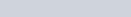Example – cube roots of 1

In this series of videos I look at a method using De Moivre’s theorem to find the nth roots of 1 by using the following example:

Find the cube roots of 1 by using De Moivre’s theorem.

I also discuss some of the graphical properties associated with this on an Argand diagram.

Complex Numbers (Cube Roots of 1, unity) : ExamSolutions Maths Video Tutorials - youtube Video

Example – 4th roots of a complex number

In this video I look at a method to find the nth roots of a complex number by using De Moivre’s theorem
In this example I find the 4th roots of a complex number by considering the solutions to this equation.

Solve the following:

1.Complex Numbers (How to find the nth root) : ExamSolutions Maths Video Tutorials - youtube Video